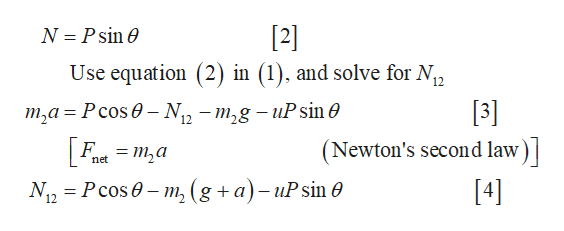# A block of mass m1 is placed on top of a block of mass m2. You push the two blocks so that they move up a vertical wall, with a constant force of magnitude P directed at angle theta.  The coefficient of friction between m2 and the wall is u. There is no friction between the blocks and no friction between m1 and the wall.  Find the force exerted by the lower block on the upper block. The answer the book gives me is (P(cos(theta)-u*sin(theta)))/(1+(m2/m1))I get how to get the numerator but am unsure of how the denominator answer was gotten and where gravity went in the equation

Question
346 views

A block of mass m1 is placed on top of a block of mass m2. You push the two blocks so that they move up a vertical wall, with a constant force of magnitude P directed at angle theta.  The coefficient of friction between m2 and the wall is u. There is no friction between the blocks and no friction between m1 and the wall.  Find the force exerted by the lower block on the upper block.

The answer the book gives me is (P(cos(theta)-u*sin(theta)))/(1+(m2/m1))

I get how to get the numerator but am unsure of how the denominator answer was gotten and where gravity went in the equation

check_circle

Step 1

Free body diagram of the mass m2 is shown below.

Step 2

Let N12 is the normal force between mass m1 and m2. From the free body diagram, write the expression for net force in the vertical direction.

Step 3

From the free body diagram, write the expression ...help_outlineImage TranscriptioncloseN Psin e  Use equation (2) in (1), and solve for N, 12  т,а %3 Рсos @ - N),, — т,g — uP sin @ 12 (Newton's second law Ftm2a net N, 3 Рcos @ - m, (g +a)-uPsin ө  fullscreen

### Want to see the full answer?

See Solution

#### Want to see this answer and more?

Solutions are written by subject experts who are available 24/7. Questions are typically answered within 1 hour.*

See Solution
*Response times may vary by subject and question.
Tagged in
SciencePhysics

### Friction